RDDtools works in an object-oriented way: the user has to define once the characteristic of the data, creating a rdd_data object, on which different anaylsis tools can be applied.

# Data Preparation and Visualisation

library(rddtools)
data(house)

Declare the data to be a rdd_data object:

house_rdd <- rdd_data(y=house$y, x=house$x, cutpoint=0)

You can now directly summarise and visualise this data:

summary(house_rdd)
#> ### rdd_data object ###
#>
#> Cutpoint: 0
#> Sample size:
#>  -Full : 6558
#>  -Left : 2740
#>  -Right: 3818
#> Covariates: no
plot(house_rdd)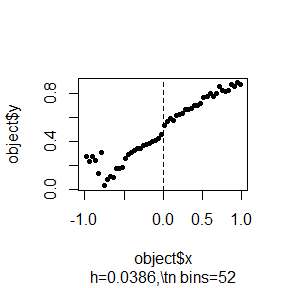# Parametric Estimation

Estimate parametrically, by fitting a 4th order polynomial.

reg_para <- rdd_reg_lm(rdd_object=house_rdd, order=4)
reg_para
#> ### RDD regression: parametric ###
#>  Polynomial order:  4
#>  Slopes:  separate
#>  Number of obs: 6558 (left: 2740, right: 3818)
#>
#>  Coefficient:
#>   Estimate Std. Error t value  Pr(>|t|)
#> D 0.076590   0.013239  5.7851 7.582e-09 ***
#> ---
#> Signif. codes:  0 '***' 0.001 '**' 0.01 '*' 0.05 '.' 0.1 ' ' 1

plot(reg_para)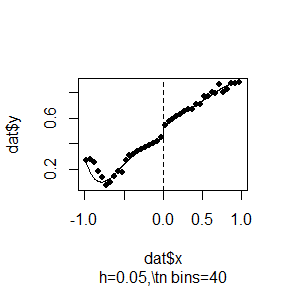# Non-parametric Estimation

Run a simple local regression, using the [Imbens and Kalyanaraman 2012] bandwidth.

bw_ik <- rdd_bw_ik(house_rdd)
reg_nonpara <- rdd_reg_np(rdd_object=house_rdd, bw=bw_ik)
print(reg_nonpara)
#> ### RDD regression: nonparametric local linear###
#>  Bandwidth:  0.2938561
#>  Number of obs: 3200 (left: 1594, right: 1606)
#>
#>  Coefficient:
#>   Estimate Std. Error z value  Pr(>|z|)
#> D 0.079924   0.009465  8.4443 < 2.2e-16 ***
#> ---
#> Signif. codes:  0 '***' 0.001 '**' 0.01 '*' 0.05 '.' 0.1 ' ' 1
plot(x=reg_nonpara)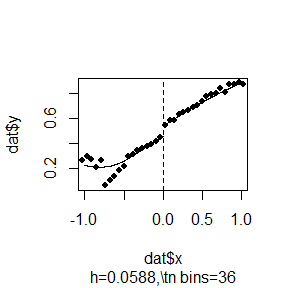# Regression Sensitivity tests:

One can easily check the sensitivity of the estimate to different bandwidths:

plotSensi(reg_nonpara, from=0.05, to=1, by=0.1)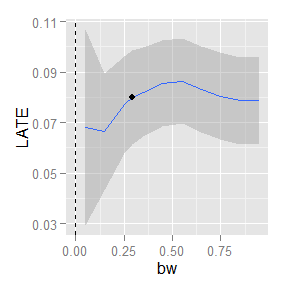Or run the Placebo test, estimating the RDD effect based on fake cutpoints:

plotPlacebo(reg_nonpara)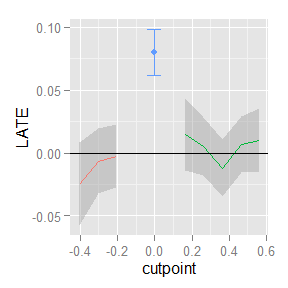# Design Sensitivity tests:

Design sensitivity tests check whether the discontinuity found can actually be attributed ot other causes. Two types of tests are available:

• Discontinuity comes from manipulation: test whether there is possible manipulation around the cutoff, McCrary 2008 test: dens_test()
• Discontinuity comes from other variables: should test whether discontinuity arises with covariates. Currently, only simple tests of equality of covariates around the threshold are available:

## Discontinuity comes from manipulation: McCrary test

use simply the function dens_test(), on either the raw data, or the regression output:

dens_test(reg_nonpara)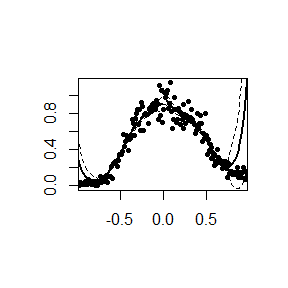#>
#>  McCrary Test for no discontinuity of density around cutpoint
#>
#> data:  reg_nonpara
#> z-val = 1.2952, p-value = 0.1952
#> alternative hypothesis: Density is discontinuous around cutpoint
#> sample estimates:
#> Discontinuity
#>     0.1035008

## Discontinuity comes from covariates: covariates balance tests

Two tests available: + equal means of covariates: covarTest_mean() + equal density of covariates: covarTest_dens()

We need here to simulate some data, given that the Lee (2008) dataset contains no covariates. We here simulate three variables, with the second having a different mean on the left and the right.

set.seed(123)
n_Lee <- nrow(house)
Z <- data.frame(z1 = rnorm(n_Lee, sd=2),
z2 = rnorm(n_Lee, mean = ifelse(house<0, 5, 8)),
z3 = sample(letters, size = n_Lee, replace = TRUE))
house_rdd_Z <- rdd_data(y = house$y, x = house$x, covar = Z, cutpoint = 0)

Run the tests:

## test for equality of means around cutoff:
covarTest_mean(house_rdd_Z, bw=0.3)
#>    mean of x   mean of y Difference statistic  p.value
#> z1 0.004268177 0.0218581 0.01758993 -0.2539109 0.7995803
#> z2 5.005608    7.984865  2.979257   -84.84982  0
#> z3 13.18888    13.43534  0.2464617  -0.9409715 0.3467888

## Can also use function covarTest_dis() for Kolmogorov-Smirnov test:
covarTest_dis(house_rdd_Z, bw=0.3)
#> Warning in ks.test(x[regime], x[!regime], exact = exact): p-value will be
#> approximate in the presence of ties
#>    statistic  p.value
#> z1 0.03482029 0.2726692
#> z2 0.8647849  0
#> z3 0.03008545 0.447416

Tests correctly reject equality of the second, and correctly do not reject equality for the first and third.